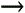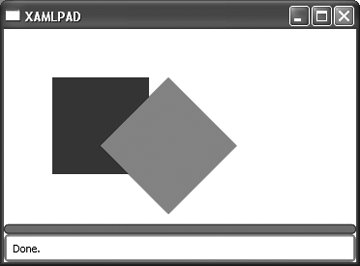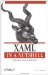# RotateTransform

 RotateTransform Hierarchy: DependencyObjectFreezableAnimatableTransform

`         <RotateTransform             Center="0,0"             Angle="45" /> `

or:

`     <RotateTransform             Angle="45" >             <RotateTransform.Center>                 <Point X="0" Y="0" />             </RotateTransform.Center>         </RotateTransform> `

<RotateTransform .../> describes a rotation around a point, based on the specified angle. Figure 11-1 shows the result of applying a 45-degree rotation to a Rectangle (Example 11-3).

##### Figure 11-1. Rectangle and rotated rectangle comparison#### Attributes

Angle (optional)

This Double value describes the angle of rotation.

Center (optional)

This Point describes the point around which the element is rotated.XAML in a Nutshell (In a Nutshell (OReilly))
ISBN: 0596526733
EAN: 2147483647
Year: 2007
Pages: 217